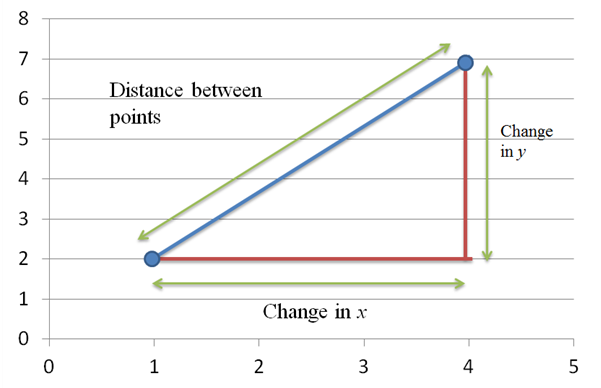# State the distance formula.### Precalculus: Mathematics for Calcu...

6th Edition
Stewart + 5 others
Publisher: Cengage Learning
ISBN: 9780840068071### Precalculus: Mathematics for Calcu...

6th Edition
Stewart + 5 others
Publisher: Cengage Learning
ISBN: 9780840068071

#### Solutions

Chapter 1, Problem 23RCC

a.

To determine

## State the distance formula.

Expert Solution

The distance formula is D=(x2x1)2+(y2y1)2 .

### Explanation of Solution

Given information:

State the distance formula.

Calculation:

Take points (x,y) on the coordinate plane and draw a line between them to determine the length of line to form a right triangle with line length equal to hypotenuse.

Graphically,Applying the pythagorean theorem to points (x1,y1) and (x2,y2) we get,

D2=(x2x1)2+(y2y1)2

Where D is the distance between the points. Solving for D gives distance formula.

D=(x2x1)2+(y2y1)2

Hence, the distance formula is D=(x2x1)2+(y2y1)2 .

b.

To determine

### State the Midpoint Formula.

Expert Solution

The midpoint formula is x=x1+x22,y=y1+y22

### Explanation of Solution

Given information:

State the Midpoint Formula.

Calculation:

Given two points on the coordinate plane are (x,y) to find the midpoint of line segment joining them by averaging the values of points (x,y) coordinates.

Points (x1,y1),(x2,y2) the midpoint formula is;

x=x1+x22,y=y1+y22

Hence, the midpoint formula is x=x1+x22,y=y1+y22

### Have a homework question?

Subscribe to bartleby learn! Ask subject matter experts 30 homework questions each month. Plus, you’ll have access to millions of step-by-step textbook answers!